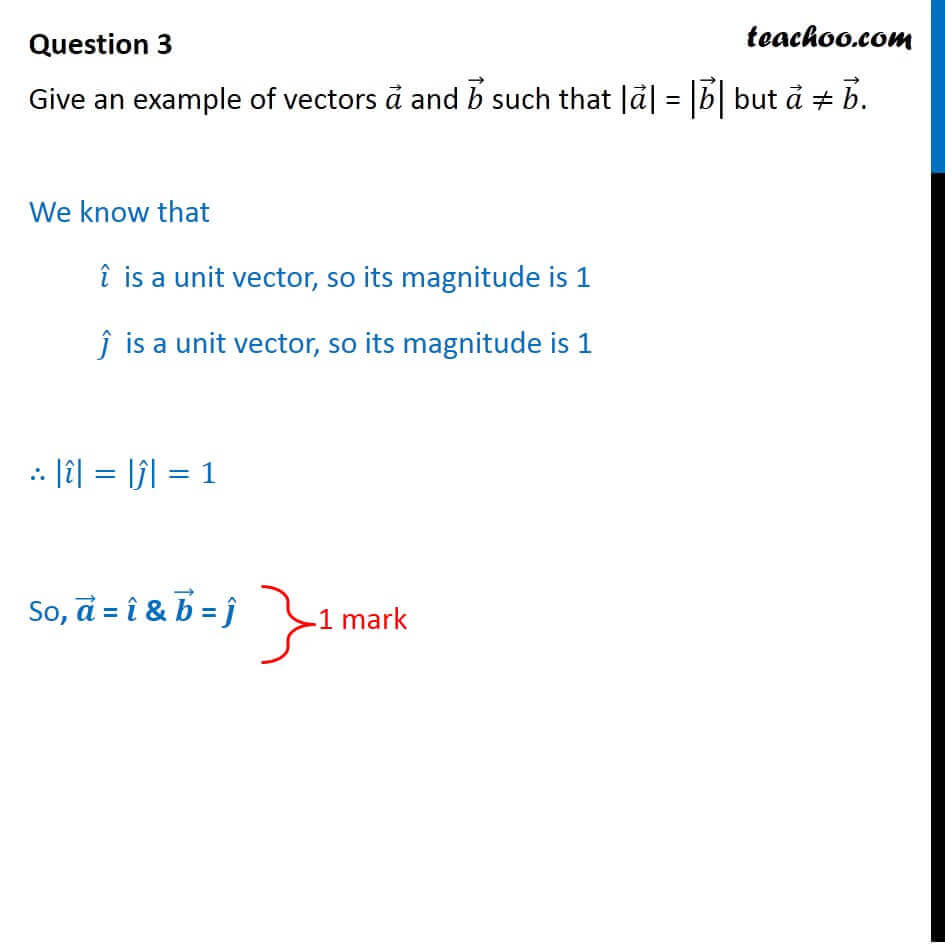CBSE Class 12 Sample Paper for 2018 Boards

Class 12
Solutions of Sample Papers and Past Year Papers - for Class 12 Boards

### Give an example of vectors a  and b  such that |a| = |b| but a ≠ b .

This is a question of CBSE Sample Paper - Class 12 - 2017/18.Get live Maths 1-on-1 Classs - Class 6 to 12

### Transcript

Question 3 Give an example of vectors 𝑎 ⃗ and 𝑏 ⃗ such that |𝑎 ⃗ | = |𝑏 ⃗ | but 𝑎 ⃗ ≠ 𝑏 ⃗. We know that 𝑖 ̂ is a unit vector, so its magnitude is 1 𝑗 ̂ is a unit vector, so its magnitude is 1 ∴ |𝑖 ̂ |=|𝑗 ̂ |=1 So, 𝒂 ⃗ = 𝒊 ̂ & 𝒃 ⃗ = 𝒋 ̂